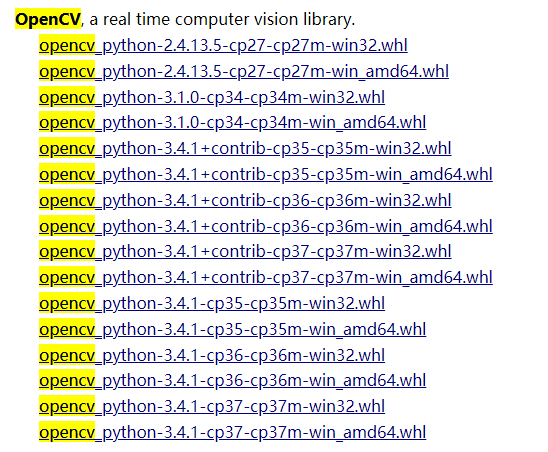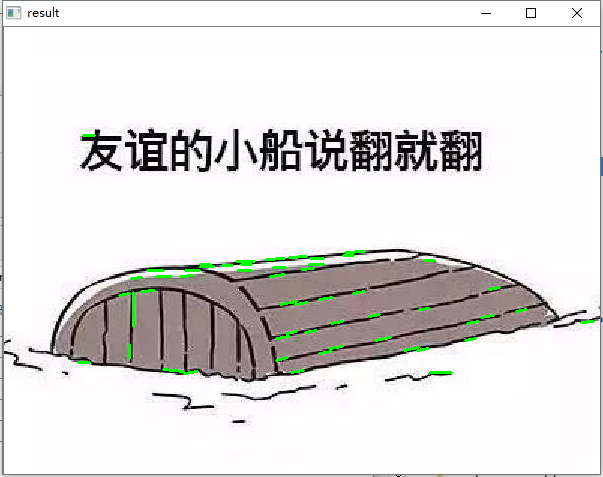• 目录：(一)原理(二)代码(标准霍夫线变换，统计概率霍夫线变换)(一)原理1....最基本的霍夫变换是从黑白图像中检测直线(线段)。2.Hough变换的原理是将特定图形上的点变换到一组参数空间上，根据参数空间...

目录：

(一)原理

(二)代码(标准霍夫线变换，统计概率霍夫线变换)

(一)原理

1.霍夫变换(Hough Transform) 霍夫变换是图像处理中从图像中识别几何形状的基本方法之一，应用很广泛，也有很多改进算法。主要用来从图像中分离出具有某种相同特征的几何形状(如，直线，圆等)。最基本的霍夫变换是从黑白图像中检测直线(线段)。

2.Hough变换的原理是将特定图形上的点变换到一组参数空间上，根据参数空间点的累计结果找到一个极大值对应的解，那么这个解就对应着要寻找的几何形状的参数(比如说直线，那么就会得到直线的斜率k与常熟b，圆就会得到圆心与半径等等)

3.霍夫线变换是一种用来寻找直线的方法。用霍夫线变换之前, 首先需要对图像进行边缘检测的处理，也即霍夫线变换的直接输入只能是边缘二值图像。

具体原理：https://blog.csdn.net/ycj9090900/article/details/52944708

(二)代码(标准霍夫线变换，统计概率霍夫线变换)

1 #直线检测

2 #使用霍夫直线变换做直线检测，前提条件：边缘检测已经完成

3 importcv2 as cv4 importnumpy as np5

6 #标准霍夫线变换

7 defline_detection(image):8 gray =cv.cvtColor(image, cv.COLOR_RGB2GRAY)9 edges = cv.Canny(gray, 50, 150, apertureSize=3) #apertureSize参数默认其实就是3

10 cv.imshow("edges", edges)11 lines = cv.HoughLines(edges, 1, np.pi/180, 80)12 for line inlines:13 rho, theta = line #line存储的是点到直线的极径和极角，其中极角是弧度表示的。

14 a = np.cos(theta) #theta是弧度

15 b =np.sin(theta)16 x0 = a * rho #代表x = r * cos(theta)

17 y0 = b * rho #代表y = r * sin(theta)

18 x1 = int(x0 + 1000 * (-b)) #计算直线起点横坐标

19 y1 = int(y0 + 1000 * a) #计算起始起点纵坐标

20 x2 = int(x0 - 1000 * (-b)) #计算直线终点横坐标

21 y2 = int(y0 - 1000 * a) #计算直线终点纵坐标 注：这里的数值1000给出了画出的线段长度范围大小

#，数值越小，画出的线段越短，数值越大，画出的线段越长

22 cv.line(image, (x1, y1), (x2, y2), (0, 0, 255), 2) #点的坐标必须是元组，不能是列表。

23 cv.imshow("image-lines", image)24

25 #统计概率霍夫线变换

26 defline_detect_possible_demo(image):27 gray =cv.cvtColor(image, cv.COLOR_RGB2GRAY)28 edges = cv.Canny(gray, 50, 150, apertureSize=3) #apertureSize参数默认其实就是3

29 lines = cv.HoughLinesP(edges, 1, np.pi / 180, 60, minLineLength=60, maxLineGap=5)30 for line inlines:31 x1, y1, x2, y2 =line32 cv.line(image, (x1, y1), (x2, y2), (0, 0, 255), 2)33 cv.imshow("line_detect_possible_demo",image)34

41 line_detect_possible_demo(src)42 cv.waitKey(0)43 cv.destroyAllWindows()

注意：

1.opencv的HoughLines函数是标准霍夫线变换函数，该函数的功能是通过一组参数对的集合来表示检测到的直线，

其函数原型为：HoughLines(image, rho, theta, threshold[, lines[, srn[, stn[, min_theta[, max_theta]]]]]) -> lines

image参数表示边缘检测的输出图像，该图像为单通道8位二进制图像。

rho参数表示参数极径以像素值为单位的分辨率，这里一般使用1像素。

theta参数表示参数极角以弧度为单位的分辨率，这里使用1度。

threshold参数表示检测一条直线所需最少的曲线交点。

lines参数表示储存着检测到的直线的参数对的容器 。

srn参数、stn参数默认都为0。如果srn = 0且stn = 0，则使用经典的Hough变换。

min_theta参数表示对于标准和多尺度Hough变换，检查线条的最小角度。

max_theta参数表示对于标准和多尺度Hough变换，检查线条的最大角度。

2.opencv的HoughLinesP函数是统计概率霍夫线变换函数，该函数能输出检测到的直线的端点其函数原型为：HoughLinesP(image, rho, theta, threshold[, lines[, minLineLength[, maxLineGap]]]) -> lines

image参数表示边缘检测的输出图像，该图像为单通道8位二进制图像。

rho参数表示参数极径以像素值为单位的分辨率，这里一般使用 1 像素。

theta参数表示参数极角以弧度为单位的分辨率，这里使用 1度。

threshold参数表示检测一条直线所需最少的曲线交点。

lines参数表示储存着检测到的直线的参数对的容器，也就是线段两个端点的坐标。

minLineLength参数表示能组成一条直线的最少点的数量，点数量不足的直线将被抛弃。

maxLineGap参数表示能被认为在一条直线上的亮点的最大距离。

参考：

https://www.cnblogs.com/FHC1994/p/9138315.html

展开全文• 语音信号的端点检测方法有很多种，简单的方法可以直接通过计算出声音的音量大小，找到音量大于某个阈值的部分，认为该部分为需要的语音信号，该部分与阈值的交点即为端点，其余部分认为非语音帧。计算音量计算音量的...

语音信号的端点检测方法有很多种，简单的方法可以直接通过计算出声音的音量大小，找到音量大于某个阈值的部分，认为该部分为需要的语音信号，该部分与阈值的交点即为端点，其余部分认为非语音帧。

计算音量

计算音量的方法有两种，一种是以帧为单位(每一帧包含多个采样点)，将该帧内的所有采样点的幅值的绝对值之后相加，作为该帧的音量值:

Vi = sum(|Wi|)

以采样率为 11025 Hz ，时长为 1s 的波形为例：该波形含有 11025 个采样点，若取帧长为 framesize = 256，帧间重叠大小为 overlap = 128，则计算出来的音量数组包含 frameNum = 11025 / (256 - 128) = 86.13，取整为 frameNum = 87。计算前 86 帧的音量代码(代码为 volume.py 的 calcNormalVolume)：

for i in range(frameNum - 1):

# 获取第 i 帧数据

curFrame = wave[i*step: i*step + framesize]

curFrame = curFrame - np.mean(curFrame)

# 公式： v = sum(|w|)

volume[i] = np.sum(np.abs(curFrame))

不论采样点的数量是否能够被帧大小整除，最后一帧都需要单独判断，取波形长度和下一帧的波形长度较小的一个，并计算最后一帧的音量：

curFrame = curFrame - np.mean(curFrame)

另一种方法是计算分贝音量，与上面的代码差别在于计算 volume 时，使用的公式不同：

Vi = 10 * log10( sum(|Wi| ^ 2) )

因此计算 volume[i] 的代码需要修改一下：

v = np.sum(np.power(curFrame, 2))

volume[i] = 10 * np.log10(v) if v > 0 else 0

一般很少出现平方和 v 的值为 0 的情况，不过为了避免这种情况，计算时当 v = 0 时不需要经过 log 运算，直接给音量赋 0 值。

理论上讲，当上面代码中计算出 v 为 0 的情况，经过对数运算后得到的值应该为 负无穷 而不是 0。

根据阈值找到端点

计算出音量后，就得到了一组离散的点，将其绘制在窗口上可以得到一个曲线， 阈值就是平行于横轴的一条直线，这条直线与曲线的交点认为是端点。判断曲线是否与阈值相交的方法很简单，(ys[i] - threshold) * (ys[i+1] - threshold) < 0。这个方法的缺点在于，当 ys[i] 或者 ys[i+1] 恰好等于 threshold 时，可能会遗漏端点。

def simpleEndPointDetection(vol, wave: vp.Wave, thresholds):

"""一种简单的端点检测方法，首先计算出声波信号的音量(能量)，分别以

音量最大值的10%和音量最小值的10倍为阈值，最后以前两种阈值的一半作为阈值。

分别找到三个阈值与波形的交点并绘制图形，在查找交点时，各个阈值之间没有相互联系

figure 1：绘制出声波信号的波形，并分别用 red green blue 三种颜色的竖直线段

画出检测到的语音信号的端点

figure 2: 绘制声波音量的波形。并分别用 red green blue 三种颜色的音量阈值横线

表示出三种不同的阈值。

"""

# 给出三个固定的阈值

threshold1 = thresholds

threshold2 = thresholds

threshold3 = thresholds

deltatime = wave.deltatime

frame = np.arange(0, len(vol)) * deltatime

# 分别找出三个不同的阈值

index1 = vp.findIndex(vol, threshold1) * deltatime

index2 = vp.findIndex(vol, threshold2) * deltatime

index3 = vp.findIndex(vol, threshold3) * deltatime

end = len(wave.ws) * (1.0 / wave.framerate)

plt.subplot(211)

plt.plot(wave.ts,wave.ws,color="black")

if len(index1) > 0:

plt.plot([index1,index1],[-1,1],'-r')

if len(index2) > 0:

plt.plot([index2,index2],[-1,1],'-g')

if len(index3) > 0:

plt.plot([index3,index3],[-1,1],'-b')

plt.ylabel('Amplitude')

plt.subplot(212)

plt.plot(frame, vol, color="black")

if len(index1) > 0:

plt.plot([0,end],[threshold1,threshold1],'-r', label="threshold 1")

if len(index2) > 0:

plt.plot([0,end],[threshold2,threshold2],'-g', label="threshold 2")

if len(index3) > 0:

plt.plot([0,end],[threshold3,threshold3],'-b', label="threshold 3")

plt.legend()

plt.ylabel('Volume(absSum)')

plt.xlabel('time(seconds)')

plt.show()

上述代码通过 findIndex 找出端点的序号 index1、index2、index3，然后计算出这几个端点在时间轴上的数值(乘以 deltatime)。最后使用 plt 进行绘制。

另一种比较复杂的是在给定一个阈值的基础上，再通过一个新的 threshold 计算出更大的语音部分，详见 volume.py 中的 findIndexWithPreIndex。这种方法于上面的类似，但是只用到了两组端点索引。

calcNormalVolume 和 calcDbVolume 计算得到的曲线不同，最终得到的端点也不一样。以 one.wav 为语音样本，通过 DbVolume 计算得到的端点效果不如 normalVolume 得到的端点。

根据过零率找到端点

计算过零率也是以帧为单位，判断每两个相邻的采样值是否异号，代码和 findIndex 类似，这样可以得到每一帧中越过 0 的采样点的个数：

zcr[i] = sum(curFrame[:-1]*curFrame[1:] < 0) / framesize

与音量曲线类似，给定阈值之后就可以找到端点。绘制出过零率后可以看到，语音部分的过零率比非语音部分的过零率要低很多。小结

不论是通过音量还是过零率，实际上都是通过固定的阈值找到端点，当信噪比较大情况下，很容易找到端点(one.wav 中的信噪比较大)，但当信噪比较小时，固定的阈值就不一定能够找到端点了。并且固定的阈值(本文中用到的是占音量区间一定比例作为阈值)并不适应不同的语音信号，难以找出端点。

其他常见的还有 MFCC 系数、自相关函数等等方法可以找到语音信号的端点。

代码

github :

参考

展开全文• 利用OpenCV-python进行直线检测

万次阅读 2018-04-29 23:48:39
最近需要利用摄像头对细小的偏移做矫正，由于之前的界面工具是用 PyQT 所写，在当前的工具中加入摄像头矫正程序，也打算用 python 直接完成。 OpenCV 简介：Python 处理图像有 OpenCV 库。OpenCV 可以运行在 Linux...

最近需要利用摄像头对细小的偏移做矫正，由于之前的界面工具是用 PyQT 所写，在当前的工具中加入摄像头矫正程序，也打算用 python 直接完成。

OpenCV 简介：

Python 处理图像有 OpenCV 库。OpenCV 可以运行在 Linux，windows，macOS 上，由 C 函数和 C++ 类构成，用于实现计算机图像、视频的编辑，应用于图像识别、运动跟踪、机器视觉等领域。

OpenCV 安装：

OpenCV 无法用 pip 或easy_install 安装，需要手动下载 .whl 文件安装。

实际应用中安装的OpenCV 库版本为 2.4.13。之所以用 2.4.13 版本的OpenCV，不采用 3.4 版本，是因为项目中用到的直线检测算法——霍夫变换，在OpenCV 3.4 中只能检测到一条结果，这条结果是所有线段中最长的一条。

从以下网址获取 OpenCV 的安装包（网页加载的速度比较慢，需要静候）

https://www.lfd.uci.edu/~gohlke/pythonlibs/

打开链接，待加载完毕后，搜索 OpenCV，可以找到如下页面根据实际安装的 python 环境，下载对应的 OpenCV 安装包。

输入命令，完成 OpenCV 的安装。

pip install opencv_python‑2.4.13.5‑cp27‑cp27m‑win_amd64.whl

OpenCV 使用：

我对图像的处理是对灰度图像进行处理，不考虑 RGB 值，为了减轻实际的计算量。OpenCV 提供的 RGB 转灰度值的接口是

gray = cv2.cvtColor(cut_img, cv2.COLOR_BGR2GRAY)

得到的灰度图像与原图的比较，如下图所示要得到图片的线段，在灰度图上继续做后续操作。对图像线段进行检测的算法，是通过算子进行边缘检测，常用算子有Prewitt 算子、sobel 算子、Laplace 算子以及 Canny 算子。这里就不对这些算子的检测结果进行详述了，因为我也不是很明白几种算子实际效果的区别。本例中使用的是 canny 算子。

OpenCV 中调用 canny 算子的方式如下：

edge = cv2.Canny(image, threshold1,threshold2[, edges[, apertureSize[, L2gradient ]]])

必要参数：

第一个参数是需要处理的原图像，该图像必须为单通道的灰度图；

第二个参数是阈值1；

第三个参数是阈值2。

其中较大的阈值2用于检测图像中明显的边缘，但一般情况下检测的效果不会那么完美，边缘检测出来是断断续续的。所以这时候用较小的第一个阈值用于将这些间断的边缘连接起来。

随后进行霍夫变换，得到线段的坐标。

霍夫变换是经典的检测直线的算法。其最初用来检测图像中的直线，同时也可以将其扩展，以用来检测图像中简单的结构。

cv2.HoughLines函数输出的是[float,float]形式的ndarray，其中每个值表示检测到的线(ρ , θ)中浮点点值的参数。在本例中，函数将通过步长为1的半径和步长为π/180的角来搜索所有可能的直线。然而用这种方法检测出来的是贯穿整张图像的直线，需要检测线段时，则要采用概率霍夫变换。

lines = cv2.HoughLinesP(edges, 1,np.pi/180, 80, minLineLength, maxLineGap)

它有两个参数：

minLineLength-线的最短长度，比这个线短的都会被忽略。

maxLineGap-两条线之间的最大间隔，如果小于此值，这两条线就会被看成一条线。

这个函数的返回值就是直线的起点和终点。

最后将找到的线段均显示在图片上，即完成直线检测。

由于图片画质因素，很多看似是直线的也未检测出是直线，还有许多散碎的直线并没有连接成长线。有待后续研究解决。附上完整代码：

import cv2
import numpy as np

cv2.imshow('origin_img', img)
height = img.shape  # 高度
width  = img.shape  # 宽度
cut_img = img

gray = cv2.cvtColor(cut_img, cv2.COLOR_BGR2GRAY)
cv2.imshow('gray_img', gray)
cv2.waitKey(0)
edges = cv2.Canny(gray, 50, 150, apertureSize=3)

lines = cv2.HoughLines(edges, 1, np.pi/180, 118)
result = cut_img.copy()
minLineLength = 30 # height/32
maxLineGap = 10 # height/40
lines = cv2.HoughLinesP(edges, 1, np.pi/180, 80, minLineLength, maxLineGap)

for x1, y1, x2, y2 in lines:
cv2.line(result, (x1, y1), (x2, y2), (0,255,0), 2)

cv2.imshow('result', result)
cv2.waitKey(0)
cv2.destroyAllWindows()

展开全文python OpenCV
• python

2020-12-04 19:48:05
本节主要是对intersect_bbox 的方法进行探究，完整的代码如下：import numpy as npimport matplotlib.pyplot as pltimport matplotlib.transforms as pboximport matplotlib.path as ppathfig = plt.figure()rect = ...

本节主要是对intersect_bbox 的方法进行探究，完整的代码如下：

import numpy as np

import matplotlib.pyplot as plt

import matplotlib.transforms as pbox

import matplotlib.path as ppath

fig = plt.figure()

rect = plt.Rectangle((-1, -1), 2, 2, facecolor="#aaaaaa")

bbox = pbox.Bbox.from_bounds(-1, -1, 2, 2)

plt.xlim(-2, 2)

plt.ylim(-3,3)

linedata = []

lines =[]

for i in range(6):

verts = (np.random.random((2, 2)) - 0.5) * 3.0

linedata.append(verts)

path = ppath.Path(verts)

line, = plt.plot(verts[:, 0], verts[:, 1], color='b')

lines.append(line)

## 用于保存到本地图片

from matplotlib.backends.backend_agg import FigureCanvasAgg as FigureCanvas

canvas = FigureCanvas(fig)

canvas.print_figure("test1.png", dpi=80)

data = zip(linedata, lines)

for dt in data:

verts = dt

path = ppath.Path(verts)

if path.intersects_bbox(bbox, False):

dt.set_color('red')

canvas = FigureCanvas(fig)

canvas.print_figure("test2.png", dpi=80)

fig.clear()

lines = []

fig = plt.figure()

rect = plt.Rectangle((-1, -1), 2, 2, facecolor="#aaaaaa")

bbox = pbox.Bbox.from_bounds(-1, -1, 2, 2)

plt.xlim(-2, 2)

plt.ylim(-3,3)

for verts in linedata:

line, = plt.plot(verts[:, 0], verts[:, 1], color='b')

lines.append(line)

data = zip(linedata, lines)

for dt in data:

verts = dt

path = ppath.Path(verts)

if path.intersects_bbox(bbox):

dt.set_color('red')

canvas = FigureCanvas(fig)

canvas.print_figure("test3.png", dpi=80)

plt.show()

展开全文• 最基本的霍夫变换是从黑白图像中检测直线(线段)。2.Hough变换的原理是将特定图形上的点变换到一组参数空间上，根据参数空间点的累计结果找到一个极大值对应的解，那么这个解就对应着要寻找的几何形状的参...
• 霍夫变换是图像处理中从图像中识别几何形状的基本方法之一，应用很广泛，也有很多改进算法。...# 使用霍夫直线变换做直线检测，前提条件：边缘检测已经完成 __author__ = "boboa" # 标准霍夫线变换 def lin...
• 最基本的霍夫变换是从黑白图像中检测直线(线段)。2.Hough变换的原理是将特定图形上的点变换到一组参数空间上，根据参数空间点的累计结果找到一个极大值对应的解，那么这个解就对应着要寻找的几何形...
• 最基本的霍夫变换是从黑白图像中检测直线(线段)。 2.Hough变换的原理是将特定图形上的点变换到一组参数空间上，根据参数空间点的累计结果找到一个极大值对应的解，那么这个解就对应着要寻找...
• 最基本的霍夫变换是从黑白图像中检测直线(线段)。2.Hough变换的原理是将特定图形上的点变换到一组参数空间上，根据参数空间点的累计结果找到一个极大值对应的解，那么这个解就对应着要寻找的几何形...
• 一个列表，每一项是一个四元组，分别是直线两个端点的坐标 37 """ 38 for line in lines: 39 for x1, y1, x2, y2 in line: 40 # 在图片上画直线 41 cv2.line(img, (x1, y1), (x2, y2), (0, ...
• Opencv-Python处理车道线检测 1.导入 我们先要找一张图片，对其进行检测. import cv2 import numpy as np from matplotlib import pyplot as plt from PIL import Image import math # 读入图像 img = cv2.imread('...python opencv 边缘检测
• 形状识别之直线检测(LSD)

千次阅读 2018-05-24 12:17:51
转自一篇基于直线检测的形状识别方法，不同于霍夫直线检测。原文网址：https://blog.csdn.net/liujiabin076/article/details/74917605LSD官网（源码下载）：http://www.ipol.im/pub/art/2012/gjmr-lsd/LSD官网（在线...形状识别 直线检测
• 基于python的车道线检测

万次阅读 多人点赞 2018-07-12 21:48:10
最近在开源社区下载了一份使用opencv在python环境中实现车道线检测的代码，研究了一下，终于有点儿看懂了，寻思着写下来，免得以后忘记了。 这个车道线检测项目原本是优达学城里无人驾驶课程中的第一个上手的项目，...车道线检测 OPENCV 霍夫直线检测 Python
• python opencv检测直线 cv2.HoughLinesP

万次阅读 多人点赞 2018-09-17 22:10:22
opencv的HoughLinesP函数是统计概率霍夫线变换函数，该函数能输出检测到的直线端点 ，其函数原型为：HoughLinesP(image, rho, theta, threshold[, lines[, minLineLength[, maxLineGap]]]) -&gt; lines ...
• 本文，介绍一下，用python-opencv模块来检测直线的方法。 工具/原料 电脑 python3.6 方法/步骤 首先，要往python编译器里面加载模块和图片。 # -*- coding: utf-8 -*- import cv2 import ...
• 通过霍夫曼直线检测检测确定指针端点和圆心计算角度，精准度高 内含报告，分析全面，有使用说明 有问题可私信
• python+opencv车道线检测(简易实现)，供大家参考，具体内容如下技术栈：python+opencv实现思路：1、canny边缘检测获取图中的边缘信息；2、霍夫变换寻找图中直线；3、绘制梯形感兴趣区域获得车前范围；4、得到并绘制...
• 在此功能中，我将介绍使用OpenCV和Python代码设置对象检测和跟踪所需的功能。使用随附的代码片段，您可以轻松设置Raspberry Pi和网络摄像头，以便制作用于物体检测的便携式图像传感器。 本文适用于任何希望在...
• 入门版的车道线检测（python+opencv）

千次阅读 多人点赞 2019-06-19 22:22:45
入门版的车道线检测参考资料 参考资料 链接1 链接2python 车道线检测 opencv
• 运行环境：python3.8， numpy=1.19.5，opencv-python=4.5.1 注意：以下内容只提供了代码与运行结果，具体原理与细节见https://zhuanlan.zhihu.com/p/54866418 一、文章目录 1、相机标定 2、视频畸形修正 3、透视变换...python 视觉检测 opencv
• import cv2 import numpy as np from matplotlib import pyplot as plt __author__ = ...直线检测 霍夫直线变换 1.霍夫变换(Hough Transform) 霍夫变换是图像处理中从图像中识别几何形状的基本方法之一， ...
• 简介： ...最基本的霍夫变换是从黑白图像中检测直线(线段)。 2.Hough变换的原理是将特定图形上的点变换到一组参数空间上，根据参数空间点的累计结果找到一个极大值对应的解，那么这个解就对应着...
• 求两点间距离 /***** 求两点间距离*****/ float getDistance(CvPoint pointO, CvPoint pointA) { float distance; distance = powf((pointO.x - pointA.x), 2) + powf((pointO.y - ...点到直线的距离:P到AB的距离python
• 但是找了很多地方却找不到关于车道线的实线虚线检测，于是通过自己的奇思妙想，想到了解决的办法，于是写下此博客，希望能帮到更多的人。 入门版的车道线检测 参考的资料 在此参考代码基础上完成的实线，虚线检测 ...opencv python
• 霍夫变换常用来在图像中提取直线和圆等几何形状,如下图所示: 在这里我使用霍夫变换主要是想来提取图像中的直线. 2.基本原理 一条直线可由两个点A=(X1,Y1)和B=(X2,Y2)确定(笛卡尔坐标) 另一方面 y=kx+q也可以写成...python 计算机视觉 opencv 算法 深度学习
• import cv2 import numpy as np # 读入图像 # img = cv2.imread(r"C:\Users\Administrator\Desktop\1.jpg") img = cv2.imread("6.jpg") # 转为灰度图像 gray = cv2.cvtColor(img, cv2...# Canny边缘检测 gray=cv2....霍夫变换 直线检测 opencv python...

python直线端点检测python 订阅